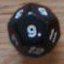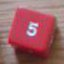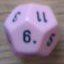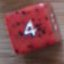incrementing inside a collection

## incrementing inside a collection

 Tue Mar 25 08:07:13 2014   by   D if I have a collection of 6 unsorted values; and I want to increment (+1) a randomly chosen value X amount of times each time independent of one another, what is the most efficient way to do that? most of the ways I've thought of run into problems letting the same value be chosen more than once. Tue Mar 25 09:30:37 2014   by   Torben I'm not exactly sure what you want to do: Do you want to increment X different values each one time or the same value X times?If it is the first you want, you can do it like this:`C := {...};  \ your initial collection of 6 valuesA := C pick X; \ pick X different values from CB := C -- A:  \ the remaining (unpicked) values from CB U (foreach v in A do v+1) \ Add to B the increments of values in A`If you want to pick one value and increment it X times, you can do it like this:`C := {...};  \ your initial collection of 6 valuesA := C pick 1; \ pick one value from CB := C -- A:  \ the remaining (unpicked) values from CB U (A+X)      \ Add (A+X) to B` Wed Mar 26 01:52:45 2014   by   D X:=3d6 C:={1,2,3,4,5,6}for every multiple of 4 that X equals or exceeds, I want to add 1 to a randomly chosen value inside C. with the choices being independent of one another. for exampleX=13 (so 3 incrementations)1) 2nd value chosen yields {1,3,3,4,5,6}2) 6th value chosen yields {1,3,3,4,5,7}3  2nd value chosen again= {1,4,3,4,5,7} Wed Mar 26 10:37:25 2014   by   Torben For this, it is best to use a function:`function increment(n,C) =  if n=0 then C  else (    A := C pick 1;    B := C -- A;    call increment(n-1, B U (A+1))  )call increment((sum 3d6)/4, {1,2,3,4,5,6})` Sun Mar 30 01:05:49 2014   by   D Is there any way to make a variable more persistent? I need to used the identical "sum 3d6" which initiates the function call value again later on for something else; and I need to use the function's modified 6 element collection output later as well. Both of which require a variable that lasts beyond the immediate function, or a very tricky arrangement of parenthesis that I have yet to figure out :P Mon Mar 31 13:05:17 2014   by   Torben I'm not exactly sure if this is what you want, but you could do something like`x := sum 3d6;y := call increment(x/4, {1,2,3,4,5,6});... something that uses x and y ...`